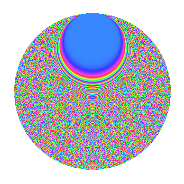# Properties

 Label 2016.2.foLevel 2016 Weight 2 Character orbit fo Rep. character $$\chi_{2016}(5,\cdot)$$ Character field $$\Q(\zeta_{24})$$ Dimension 3040 Sturm bound 768

# Related objects

## Defining parameters

 Level: $$N$$ $$=$$ $$2016 = 2^{5} \cdot 3^{2} \cdot 7$$ Weight: $$k$$ $$=$$ $$2$$ Character orbit: $$[\chi]$$ $$=$$ 2016.fo (of order $$24$$ and degree $$8$$) Character conductor: $$\operatorname{cond}(\chi)$$ $$=$$ $$2016$$ Character field: $$\Q(\zeta_{24})$$ Sturm bound: $$768$$

## Dimensions

The following table gives the dimensions of various subspaces of $$M_{2}(2016, [\chi])$$.

Total New Old
Modular forms 3104 3104 0
Cusp forms 3040 3040 0
Eisenstein series 64 64 0

## Trace form

 $$3040q - 12q^{3} - 8q^{4} - 12q^{5} - 4q^{7} - 4q^{9} + O(q^{10})$$ $$3040q - 12q^{3} - 8q^{4} - 12q^{5} - 4q^{7} - 4q^{9} - 24q^{10} - 12q^{11} - 12q^{12} - 12q^{14} - 8q^{16} - 4q^{18} - 24q^{19} - 8q^{21} - 8q^{22} - 12q^{23} - 12q^{24} + 4q^{25} - 16q^{28} - 24q^{29} + 28q^{30} - 24q^{33} - 24q^{34} + 24q^{36} - 8q^{37} - 12q^{38} - 4q^{39} - 12q^{40} + 52q^{42} - 8q^{43} + 132q^{44} - 12q^{45} - 8q^{46} - 24q^{50} - 28q^{51} - 12q^{52} - 12q^{54} - 12q^{56} - 16q^{57} + 4q^{58} - 24q^{59} + 12q^{60} + 48q^{62} - 16q^{63} - 32q^{64} - 12q^{66} - 8q^{67} - 12q^{68} - 4q^{70} - 4q^{72} - 24q^{73} - 12q^{74} - 12q^{75} - 12q^{77} - 92q^{78} - 24q^{82} - 120q^{83} + 28q^{84} - 28q^{85} - 12q^{86} - 12q^{87} + 4q^{88} - 216q^{90} - 16q^{91} - 24q^{92} + 44q^{93} - 12q^{96} - 228q^{98} - 16q^{99} + O(q^{100})$$

## Decomposition of $$S_{2}^{\mathrm{new}}(2016, [\chi])$$ into newform subspaces

The newforms in this space have not yet been added to the LMFDB.

## Hecke characteristic polynomials

There are no characteristic polynomials of Hecke operators in the database